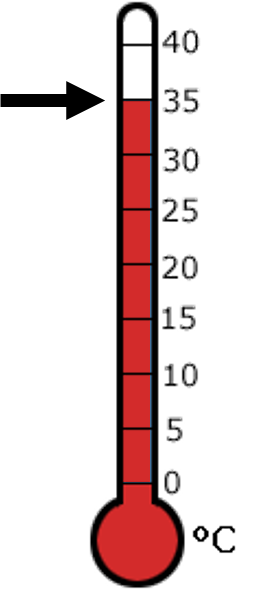1
visibility

What is the temperature shown on the given thermometer?• \$latex 50^\circ C\$

• \$latex 35^\circ C\$

• \$latex 20^\circ C\$

• \$latex 25^\circ C\$

The correct answer is  35°C.

Solution:

First, we find the point where the thermometer changes from red to white.Then, we look at the number to the right.

The temperature is  35°C  as desired.